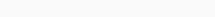# Math Puzzles Puzzle 12 Answer AndroidMath puzzle game level 12 solution with detailed hints and explanation, Scroll below to find out.

Math puzzles increase your logical thinking. The objective of the game is to find patterns hidden in the puzzle in order to arrive at the solution. Challenge yourself with different levels of math puzzles and stretch the limits of your intelligence.

### Math Puzzles Puzzle 12:

1,2=23

2,3=65

3,4=127

4,5=209

5,6=?Solution/Hint: the first digit is the multiplication of given numbers,

the last digit is the addition of numbers.

(5*6)(5+6)=3011

If there is any Doubt or you are unable to understand the solution, then please let me know in comments, I will surely help you.

### One thought on “Math Puzzles Game Level 12 Answer with Solution”

•June 30, 2019 at 14:35